Next: 5.2.8 Neighbor Links Arrays Up: 5.2 Ray Tracing Previous: 5.2.6 Spatial Subdivision

## 5.2.7 Splitting Strategies

Different splitting strategies have been proposed to reduce the average number of traversed boxes [39,72]. Among the simplest are the spatial median (SM) and the object median (OM) splitting strategy. The first algorithm chooses the splitting plane in such a way that the box is separated into two boxes of approximately equal size. The second algorithm uses the OM instead, which attempts to create boxes containing approximately the same number of objects. The objects are in this case the non-empty cells. Figure 5.3 shows the corresponding spatial subdivisions for both strategies.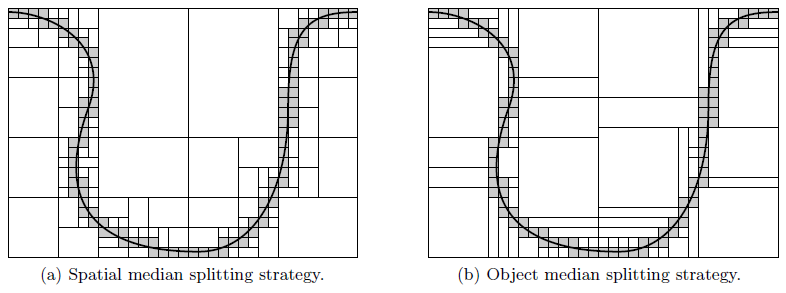A much better strategy was presented in , which explicitly attempts to reduce the average number of traversed subboxes. The surface area heuristic (SAH) strategy uses a cost model to decide which splitting plane is best. For the following considerations all rays are assumed to be uniformly distributed. This corresponds to an arriving flux distribution at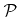, which obeys the most frequently used directional distribution, the cosine distribution (2.5). Furthermore, the cost for a complete traversal through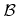is considered, which is not the case in reality, because particles are only tracked, until they reach the surface.

According to geometric probability theory , a uniformly distributed ray which intersects, also intersects another axis-aligned box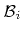withwith a probability of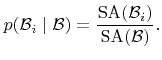(5.22)

Here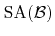and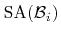denote the surface areas of boxesand, respectively. Hence the average number of traversed subboxes is given by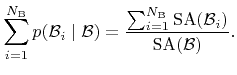(5.23)

The SAH strategy attempts to choose the splitting planes in such a way that this expression is minimized. Finding the absolute minimum of (5.23) is usually not possible in reasonable time. Instead, before subdividing a box, the additional costs are estimated in order to choose the best splitting plane.

Consider a box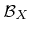during setup of the spatial subdivision, which needs to be split further. This is the case, ifis still larger than a grid cell and contains at least one non-empty cell. Let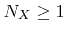be the number of non-empty grid cells inwhich is subdivided along a certain plane into boxes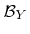and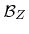, which then contain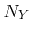and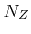(with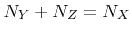) non-empty grid cells, respectively. The additional costs are reduced, if the expression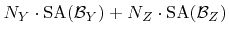(5.24)

is minimized [39,72,134]. Due to the restriction that boxes are only split along grid planes, it is possible to evaluate (5.24) for all potential splitting planes and to select the splitting plane with the minimum value. If the extent of boxin the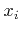-direction (in terms of grid spacings) is denoted by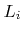, the number of potential splitting planes is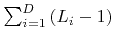. Subdivisions are favorable, which result in empty subboxes, because they do not need to be split further. For that purpose, expression (5.24) can be multiplied by a constant weight factor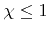, if and only if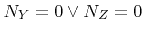is true for the corresponding splitting plane .

For good splitting strategies, such as the SAH, a logarithmic scaling with the number of objects can be observed for the average number of traversed boxes (5.23) . Here the objects correspond to the non-empty cells which scale linearly with the surface size. Hence, as already stated in Section 5.2.4, the expected computational complexity of ray tracing is in the order ofwhere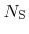is a measure of the surface size.Next: 5.2.8 Neighbor Links Arrays Up: 5.2 Ray Tracing Previous: 5.2.6 Spatial Subdivision

Otmar Ertl: Numerical Methods for Topography Simulation